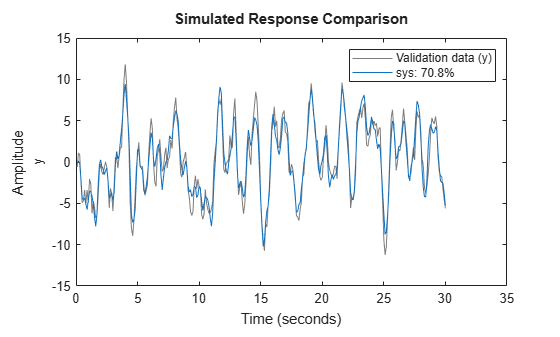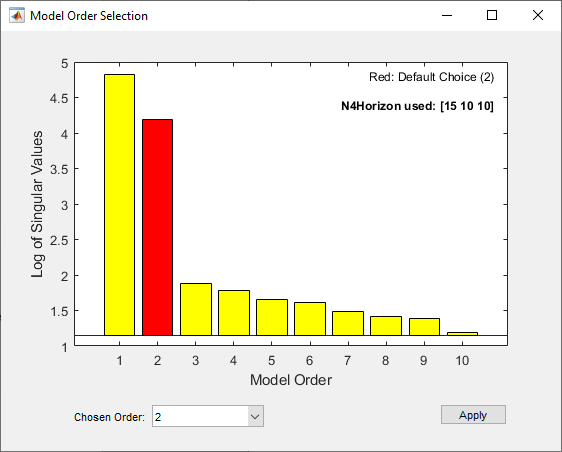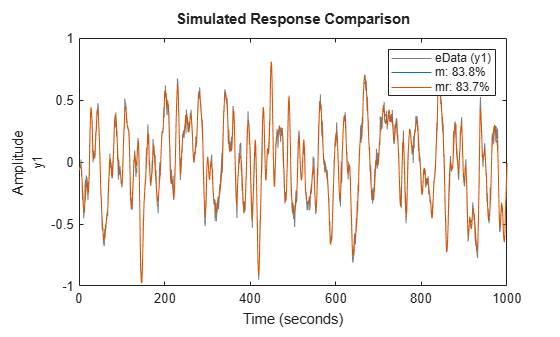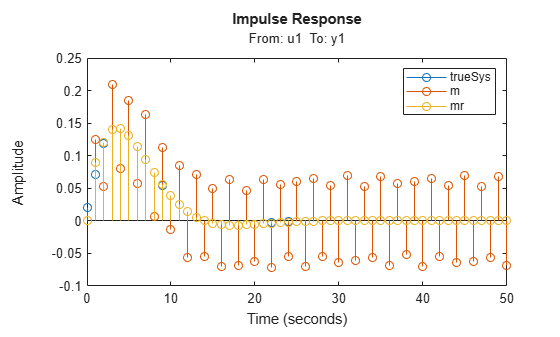# ssest

Estimate state-space model using time-domain or frequency-domain data

## Syntax

``sys = ssest(data,nx)``
``sys = ssest(data,nx,Name,Value)``
``sys = ssest(___,opt)``
``sys = ssest(data,init_sys)``
``sys = ssest(data,init_sys,opt)``
``[sys,x0] = ssest(___)``

## Description

### Estimate a State-Space Model

example

````sys = ssest(data,nx)` estimates a continuous-time state-space model `sys` of order `nx`, using data `data` that can be in the time domain or the frequency domain. `sys` is a model of the following form: $\begin{array}{l}\stackrel{˙}{x}\left(t\right)=Ax\left(t\right)+Bu\left(t\right)+Ke\left(t\right)\\ y\left(t\right)=Cx\left(t\right)+Du\left(t\right)+e\left(t\right)\end{array}$A, B, C, D, and K are state-space matrices. u(t) is the input, y(t) is the output, e(t) is the disturbance, and x(t) is the vector of `nx` states.All entries of A, B, C, and K are free estimable parameters by default. D is fixed to zero by default, meaning that there is no feedthrough, except for static systems (`nx = 0`).```

example

````sys = ssest(data,nx,Name,Value)` incorporates additional options specified by one or more name-value pair arguments. For example, estimate a discrete-time model by specifying the sample time `'Ts'` name-value pair argument. Use the `'Form'`, `'Feedthrough'`, and `'DisturbanceModel'` name-value pair arguments to modify the default behavior of the A, B, C, D, and K matrices.```

example

````sys = ssest(___,opt)` incorporates an option set `opt` that specifies options such as estimation objective, handling of initial conditions, regularization, and numerical search method used for estimation. You can specify `opt` after any of the previous input-argument combinations.```

### Configure Initial Parameters

example

````sys = ssest(data,init_sys)` uses the state-space model `init_sys` to configure the initial parameterization.```
````sys = ssest(data,init_sys,opt)` estimates the model using an option set `opt`.```

### Return Estimated Initial States

example

````[sys,x0] = ssest(___)` returns the value of initial states computed during estimation. You can use this syntax with any of the previous input-argument combinations.```

## Examples

collapse all

Estimate a state-space model and compare its response with the measured output.

Load the input-output data, which is stored in an `iddata` object.

`load iddata1 z1`

Estimate a fourth-order state-space model.

```nx = 4; sys = ssest(z1,nx);```

Compare the simulated model response with the measured output.

`compare(z1,sys)`The plot shows that the fit percentage between the simulated model and the estimation data is greater than 70%.

You can view more information about the estimation by exploring the `idss` property `sys.Report`.

`sys.Report`
```ans = Status: 'Estimated using SSEST with prediction focus' Method: 'SSEST' InitialState: 'zero' N4Weight: 'CVA' N4Horizon: [6 10 10] Fit: [1x1 struct] Parameters: [1x1 struct] OptionsUsed: [1x1 idoptions.ssest] RandState: [] DataUsed: [1x1 struct] Termination: [1x1 struct] ```

`sys.Report.Termination`
```ans = struct with fields: WhyStop: 'No improvement along the search direction with line search.' Iterations: 7 FirstOrderOptimality: 85.9759 FcnCount: 123 UpdateNorm: 9.7655 LastImprovement: 0 ```

The report includes information on the number of iterations and the reason the estimation stopped iterating.

Load the input-output data `z1`, which is stored in an `iddata` object. This is the same data used to estimate a fourth-order model in State-Space Model.

`load iddata1 z1`

Determine the optimal model order by specifying argument `nx` as a range from `1:10`.

```nx = 1:10; sys = ssest(z1,nx);```

An automatically generated plot shows the Hankel singular values for models of the orders specified by `nx`.States with relatively small Hankel singular values can be safely discarded. The suggested default order choice is `2`.

Select the model order in the Chosen Order list and click .

`load iddata7 z7;`

Identify a fourth-order state-space model of the data. Specify a known delay of `2` seconds for the first input and `0` seconds for the second input.

```nx = 4; sys = ssest(z7(1:300),nx,'InputDelay',[2;0]);```

Modify the canonical form of the A, B, and C matrices, include a feedthrough term in the D matrix, and eliminate disturbance-model estimation in the K matrix.

Load input-output data and estimate a fourth-order system using the `ssest` default options.

```load iddata1 z1 sys1 = ssest(z1,4);```

Specify the companion form and compare the `A` matrix with the default `A` matrix.

```sys2 = ssest(z1,4,'Form','companion'); A1 = sys1.A```
```A1 = 4×4 -0.5155 -3.8483 0.6657 -0.2666 5.8665 -2.7285 1.0649 -1.4694 -0.4487 0.9308 -0.6235 18.8148 -0.4192 0.5595 -16.0688 0.5399 ```
`A2 = sys2.A`
```A2 = 4×4 103 × 0 0 0 -7.1122 0.0010 0 0 -0.9547 0 0.0010 0 -0.3263 0 0 0.0010 -0.0033 ```

Include a feedthrough term and compare `D` matrices.

```sys3 = ssest(z1,4,'Feedthrough',1); D1 = sys1.D```
```D1 = 0 ```
`D3 = sys3.D`
```D3 = 0.0339 ```

Eliminate disturbance modeling and compare `K` matrices.

```sys4 = ssest(z1,4,'DisturbanceModel','none'); K1 = sys1.K```
```K1 = 4×1 0.0520 0.0973 0.0151 0.0270 ```
`K4 = sys4.K`
```K4 = 4×1 0 0 0 0 ```

Specify `ssest` estimate initial states as independent estimation parameters.

`ssest` can handle initial states using one of several methods. By default, `ssest` chooses the method automatically based on your estimation data. You can choose the method yourself by modifying the option set using `ssestOptions`.

Load the input-output data `z1` and estimate a second-order state-space model `sys` using the default options. Use the syntax that returns initial states `x0`.

```load iddata1 z1 [sys,x0] = ssest(z1,2); x0```
```x0 = 2×1 0 0 ```

By default, the estimation is performed using the `'auto'` setting for `InitialState`. Find out which method `ssest` applied by reviewing the value of `InitialState` in `sys.Repor`t.

`sys.Report.InitialState`
```ans = 'zero' ```

The software applied the '`zero'` method, meaning that the software set the initial states to zero instead of estimating them. This selection is consistent with the `0` values returned for `x0`.

Specify that `ssest` estimate the initial states instead as independent parameters using the `'estimate'` setting. Use `ssestOptions` to create a modified option set and specify that option set to estimate a new model.

```opt = ssestOptions('InitialState','estimate'); [sys1,x0] = ssest(z1,2,opt); x0```
```x0 = 2×1 0.0068 0.0052 ```

`x0` now has estimated parameters with nonzero values.

Obtain a regularized fifth-order state-space model for a second-order system from a narrow bandwidth signal.

`load regularizationExampleData eData;`

Create the transfer function model used for generating the estimation data (true system).

`trueSys = idtf([0.02008 0.04017 0.02008],[1 -1.561 0.6414],1);`

Estimate an unregularized state-space model.

```opt = ssestOptions('SearchMethod','lm'); m = ssest(eData,5,'form','modal','DisturbanceModel','none','Ts',eData.Ts,opt);```

Estimate a regularized state-space model.

```opt.Regularization.Lambda = 10; mr = ssest(eData,5,'form','modal','DisturbanceModel','none','Ts',eData.Ts,opt);```

Compare the model outputs with the estimation data.

`compare(eData,m,mr);`Compare the model impulse responses.

```impulse(trueSys,m,mr,50); legend('trueSys','m','mr');```Estimate a state-space model of measured input-output data. Configure the parameter constraints and initial values for estimation using a state-space model.

Create an `idss` model to specify the initial parameterization for estimation.

```A = blkdiag([-0.1 0.4; -0.4 -0.1],[-1 5; -5 -1]); B = [1; zeros(3,1)]; C = [1 1 1 1]; D = 0; K = zeros(4,1); x0 = [0.1 0.1 0.1 0.1]; Ts = 0; init_sys = idss(A,B,C,D,K,x0,Ts);```

Setting all entries of `K` to `0` creates an `idss` model with no state disturbance element.

Use the `Structure` property to fix the values of some of the model parameters. Configure the model so that `B` and `K` are fixed, and only the nonzero entries of `A` are estimable.

```init_sys.Structure.A.Free = (A~=0); init_sys.Structure.B.Free = false; init_sys.Structure.K.Free = false;```

The entries in `init_sys.Structure.A.Free` determine whether the corresponding entries in `init_sys.A` are free (`true`) or fixed (`false`).

Load the measured data and estimate a state-space model using the parameter constraints and initial values specified by `init_sys`.

```load iddata2 z2; sys = ssest(z2,init_sys);```

The estimated parameters of `sys` satisfy the constraints specified by `init_sys`.

## Input Arguments

collapse all

Estimation data, specified as an `iddata` object, an `frd` object, or an `idfrd` object.

For time-domain estimation, `data` must be an `iddata` object containing the input and output signal values.

For frequency-domain estimation, `data` can be one of the following:

• Recorded frequency response data (`frd` (Control System Toolbox) or `idfrd`)

• `iddata` object with properties specified as follows.

• `InputData` — Fourier transform of the input signal

• `OutputData` — Fourier transform of the output signal

• `Domain``'Frequency'`

Estimation data must be uniformly sampled. By default, the software sets the sample time of the model to the sample time of the estimation data.

For multiexperiment data, the sample times and intersample behavior of all the experiments must match.

The domain of your data determines the type of model you can estimate.

• Time-domain or discrete-time frequency-domain data — Continuous-time and discrete-time models

• Continuous-time frequency-domain data — Continuous-time models only

Order of the estimated model, specified as a nonnegative integer or as a vector containing a range of positive integers.

• If you already know what order you want your estimated model to have, specify `nx` as a scalar.

• If you want to compare a range of potential orders to choose the most effective order for your estimated model, specify the range in `nx`. `ssest` creates a Hankel singular-value plot that shows the relative energy contributions of each state in the system. States with relatively small Hankel singular values contribute little to the accuracy of the model and can be discarded with little impact. The index of the highest state you retain is the model order. The plot window includes a suggestion for the order to use. You can accept this suggestion or enter a different order. For an example, see Determine Optimal Estimated Model Order.

If you do not specify `nx`, or if you specify `nx` as `best`, the software automatically chooses `nx` from the range `1:10`.

• If you are identifying a static system, set `nx` to `0`.

Estimation options, specified as an `ssestOptions` option set. Options specified by `opt` include:

• Estimation objective

• Handling of initial conditions

• Regularization

• Numerical search method used for estimation

For examples showing how to use `opt`, see Estimate Initial States as Independent Parameters and Estimate State-Space Model Using Regularization.

Linear system that configures the initial parameterization of `sys`, specified as an `idss` model or as a structure. You obtain `init_sys` by either performing an estimation using measured data or by direct construction.

If `init_sys` is an `idss` model, `ssest` uses the parameter values of `init_sys` as the initial guess for estimating `sys`. For information on how to specify `idss`, see Estimate State-Space Models with Structured Parameterization. `ssest` honors constraints on the parameters of `init_sys`, such as fixed coefficients and minimum/maximum bounds.

Use the `Structure` property of `init_sys` to configure initial parameter values and constraints for the A, B, C, D, and K matrices. For example:

• To specify an initial guess for the A matrix of `init_sys`, set `init_sys.Structure.A.Value` as the initial guess.

• To specify constraints for the B matrix of `init_sys`:

• Set `init_sys.Structure.B.Minimum` to the minimum B matrix value

• Set `init_sys.Structure.B.Maximum` to the maximum B matrix value

• Set `init_sys.Structure.B.Free` to indicate if entries of the B matrix are free parameters for estimation

To set more complex constraints, such as interdependence of coefficients, use grey-box estimation using `greyest` and `idgrey`.

You must assign finite initial values for all matrix parameters.

If `init_sys` is not a state-space (`idss`) model, the software first converts `init_sys` to an `idss` model. `ssest` uses the parameters of the resulting model as the initial guess for estimation.

If you do not specify `opt` and `init_sys` was obtained by estimation, then the software uses estimation options from `init_sys.Report.OptionsUsed`.

For an example, see Estimate Partially Known State-Space Model Using Structured Estimation.

### Name-Value Arguments

Specify optional pairs of arguments as `Name1=Value1,...,NameN=ValueN`, where `Name` is the argument name and `Value` is the corresponding value. Name-value arguments must appear after other arguments, but the order of the pairs does not matter.

Before R2021a, use commas to separate each name and value, and enclose `Name` in quotes.

Example: `sys = ssest(data,nx,'Ts',0.1)`

Sample time of the estimated model, specified as the comma-separated pair consisting of `'Ts'` and either `0` or a positive scalar.

• For continuous-time models, specify `'Ts'` as `0`.

• For discrete-time models, specify `'Ts'` as the data sample time in the units stored in the `TimeUnit` property.

Input delay for each input channel, specified as the comma-separated pair consisting of `'InputDelay'` and a numeric vector.

• For continuous-time models, specify `'InputDelay'` in the time units stored in the `TimeUnit` property.

• For discrete-time models, specify `'InputDelay'` in integer multiples of the sample time `Ts`. For example, setting `'InputDelay'` to `3` specifies a delay of three sampling periods.

For a system with Nu inputs, set `InputDelay` to an Nu-by-1 vector. Each entry of this vector is a numerical value that represents the input delay for the corresponding input channel. For an example, see Identify State-Space Model with Input Delay.

To apply the same delay to all channels, specify `InputDelay` as a scalar.

Type of canonical form of `sys`, specified as the comma-separated pair consisting of `'Form'` and one of the following values:

• `'free'` — All entries of the matrices A, B, C, D, and K are treated as free.

• `'modal'` — Obtain `sys` in modal form.

• `'companion'` — Obtain `sys` in companion form.

• `'canonical'` — Obtain `sys` in the observability canonical form.

For definitions of the canonical forms, see Canonical State-Space Realizations.

For more information, see Estimate State-Space Models with Canonical Parameterization. For an example, see Modify Form, Feedthrough, and Disturbance-Model Matrices.

Direct feedthrough from input to output, specified as the comma-separated pair consisting of `'Feedthrough'` and a logical vector of length Nu, where Nu is the number of inputs. If you specify `Feedthrough` as a logical scalar, that value is applied to all the inputs. For static systems, the software always assumes `'Feedthrough'` is `1`.

For an example, see Modify Form, Feedthrough, and Disturbance-Model Matrices.

Option to estimate time-domain noise component parameters in the K matrix, specified as the comma-separated pair consisting of `'DisturbanceModel'` and one of the following values:

• `'estimate'` — Estimate the noise component. The K matrix is treated as a free parameter.

• `'none'` — Do not estimate the noise component. The elements of the K matrix are fixed at zero.

For frequency-domain data, the software assumes that `'DisturbanceModel'` is `'none'`.

For an example, see Modify Form, Feedthrough, and Disturbance-Model Matrices.

## Output Arguments

collapse all

Identified state-space model, returned as an `idss` model. This model is created using the specified model orders, delays, and estimation options.

Information about the estimation results and options used is stored in the `Report` property of the model. `Report` has the following fields.

Report FieldDescription
`Status`

Summary of the model status, which indicates whether the model was created by construction or obtained by estimation.

`Method`

Estimation command used.

`InitialState`

How initial states were handled during estimation, returned as one of the following values:

• `'zero'` — The initial state is set to zero.

• `'estimate'` — The initial state is treated as an independent estimation parameter.

• `'backcast'` — The initial state is estimated using the best least squares fit.

• Column vector of length Nx, where Nx is the number of states. For multi-experiment data, a matrix with Ne columns, where Ne is the number of experiments.

• Parametric initial condition object (`x0obj`) created using `idpar`. Only for discrete-time state-space models.

This field is especially useful when the `InitialState` option in the estimation option set is `'auto'`.

`N4Weight`

Weighting scheme used for singular-value decomposition by the N4SID algorithm, returned as one of the following values:

• `'MOESP'` — Uses the MOESP algorithm.

• `'CVA'` — Uses the Canonical Variate Algorithm.

• `'SSARX'` — A subspace identification method that uses an ARX estimation-based algorithm to compute the weighting.

This option is especially useful when the `N4Weight` option in the estimation option set is `'auto'`.

`N4Horizon`

Forward and backward prediction horizons used by the N4SID algorithm, returned as a row vector with three elements — ` [r sy su]`, where `r` is the maximum forward prediction horizon. `sy` is the number of past outputs, and `su` is the number of past inputs that are used for the predictions.

`Fit`

Quantitative assessment of the estimation, returned as a structure. See Loss Function and Model Quality Metrics for more information on these quality metrics. The structure has the following fields:

FieldDescription
`FitPercent`

Normalized root mean squared error (NRMSE) measure of how well the response of the model fits the estimation data, expressed as the percentage fitpercent = 100(1-NRMSE).

`LossFcn`

Value of the loss function when the estimation completes.

`MSE`

Mean squared error (MSE) measure of how well the response of the model fits the estimation data.

`FPE`

Final prediction error for the model.

`AIC`

Raw Akaike Information Criteria (AIC) measure of model quality.

`AICc`

Small-sample-size corrected AIC.

`nAIC`

Normalized AIC.

`BIC`

Bayesian Information Criteria (BIC).

`Parameters`

Estimated values of model parameters.

`OptionsUsed`

Option set used for estimation. If no custom options were configured, this is a set of default options. See `ssestOptions` for more information.

`RandState`

State of the random number stream at the start of estimation. Empty, `[]`, if randomization was not used during estimation. For more information, see `rng`.

`DataUsed`

Attributes of the data used for estimation. Structure with the following fields:

FieldDescription
`Name`

Name of the data set.

`Type`

Data type.

`Length`

Number of data samples.

`Ts`

Sample time. This is equivalent to `Data.Ts`.

`InterSample`

Input intersample behavior. One of the following values:

• `'zoh'` — Zero-order hold maintains a piecewise-constant input signal between samples.

• `'foh'` — First-order hold maintains a piecewise-linear input signal between samples.

• `'bl'` — Band-limited behavior specifies that the continuous-time input signal has zero power above the Nyquist frequency.

The value of `Intersample` has no effect on estimation results for discrete-time models.

`InputOffset`

Offset removed from time-domain input data during estimation.

`OutputOffset`

Offset removed from time-domain output data during estimation.

`Termination`

Termination conditions for the iterative search used for prediction error minimization, returned as a structure with the following fields:

FieldDescription
`WhyStop`

Reason for terminating the numerical search.

`Iterations`

Number of search iterations performed by the estimation algorithm.

`FirstOrderOptimality`

$\infty$-norm of the gradient search vector when the search algorithm terminates.

`FcnCount`

Number of times the objective function was called.

`UpdateNorm`

Norm of the gradient search vector in the last iteration. Omitted when the search method is `'lsqnonlin'` or `'fmincon'`.

`LastImprovement`

Criterion improvement in the last iteration, expressed as a percentage. Omitted when the search method is `'lsqnonlin'` or `'fmincon'`.

`Algorithm`

Algorithm used by `'lsqnonlin'` or `'fmincon'` search method. Omitted when other search methods are used.

For estimation methods that do not require numerical search optimization, the `Termination` field is omitted.

For more information on using `Report`, see Estimation Report.

Initial states computed during the estimation, returned as an array containing a column vector corresponding to each experiment.

This array is also stored in the `Parameters` field of the model `Report` property.

For an example, see Estimate Initial States as Independent Parameters.

## Algorithms

`ssest` initializes the parameter estimates using either a noniterative subspace approach or an iterative rational function estimation approach. It then refines the parameter values using the prediction error minimization approach. For more information, see `pem` and `ssestOptions`.

 Ljung, L. System Identification: Theory for the User, Second Edition. Upper Saddle River, NJ: Prentice Hall PTR, 1999.